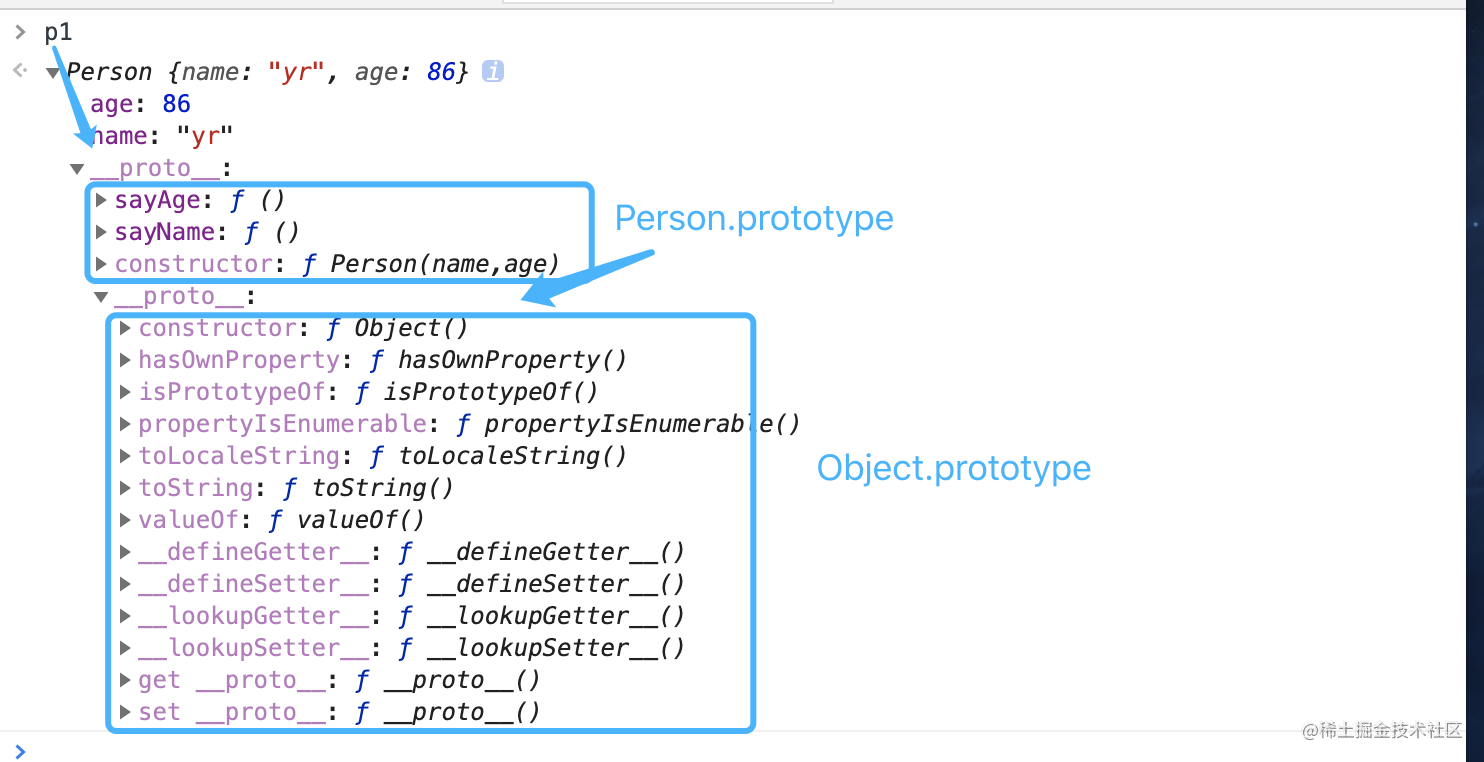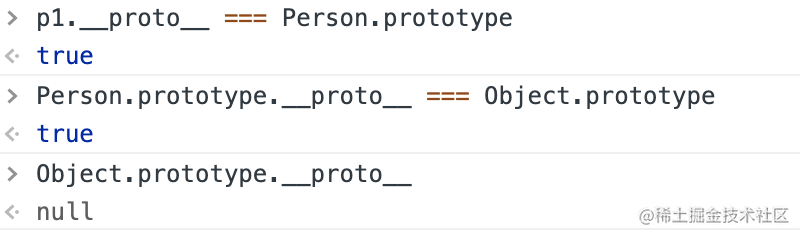js的原型与原型链

工厂模式

function createPeople(name,age){
var o = {};
o.name = name;
o.age = age;
o.sayName = function(){
console.log(this.name)
}
return o;
}
let p1 = createPeople('yr', 18);
let p2 = createPeople('lyl', 22);
p1.sayName(); //yr
p2.sayName(); //lyl

构造函数

function Person(name,age){
this.name = name;
this.age = age;
this.sayName = function(){
console.log(this.name)
}
}
let p1 = new Person('yr',86);
let p2 = new Person('lyl',88);

function Person(name,age){
this.name = name;
this.age = age;
this.sayName = function(){
console.log(this.name)
}
}
Person('yr',86);//添加到window
console.log(window.sayName());//yr

new关键字

1. 创建一个新对象
2. 将构造函数的作用域赋值给新对象(this指向新对象)
3. 执行构造函数中的代码(为这个新对象添加属性)
4. 返回新对象

p1和p2分别保存着Person的一个不同实例，这两个对象有一个constructor(构造函数)属性，都指向Person。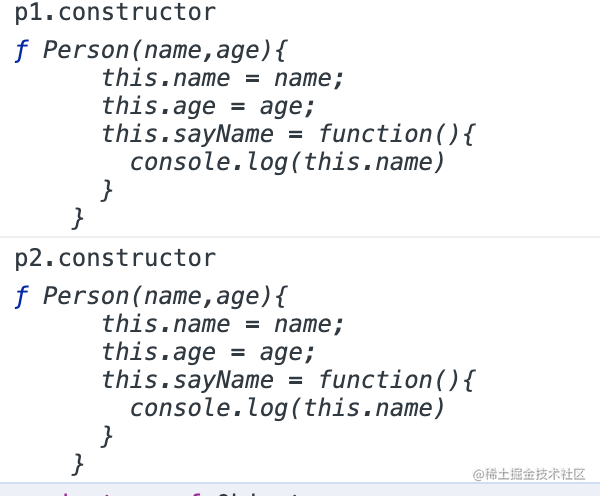constructor属性最初是用来标识对象类型的，但是，检测对象类型，还是需要用instanceof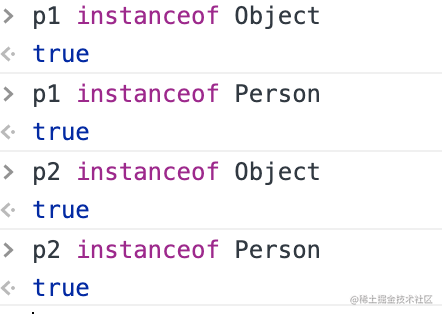p1和p2都是Object的实例，是因为所有对象都继承自Object

原型实例

function Person(name,age){
this.name = name;
this.age = age;
}
Person.prototype.sayName = function(){
console.log(this.name)
}
let p1 = new Person('yr',86);
let p2 = new Person('lyl',88);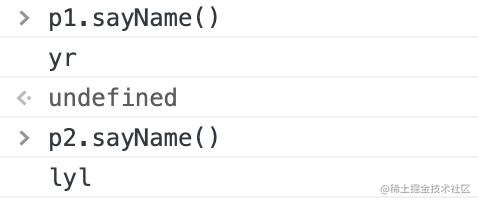理解原型对象

isPrototypeOfgetPrototypeOf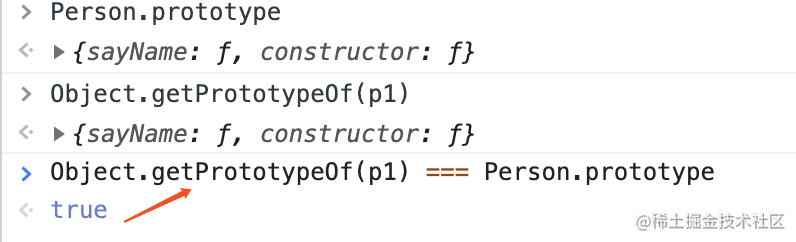hasOwnPrototype

function Person(age){
this.age = age;
}
Person.prototype.name = 'yr'
Person.prototype.sayName = function(){
console.log(this.name)
}
let p1 = new Person(86);
let p2 = new Person(88);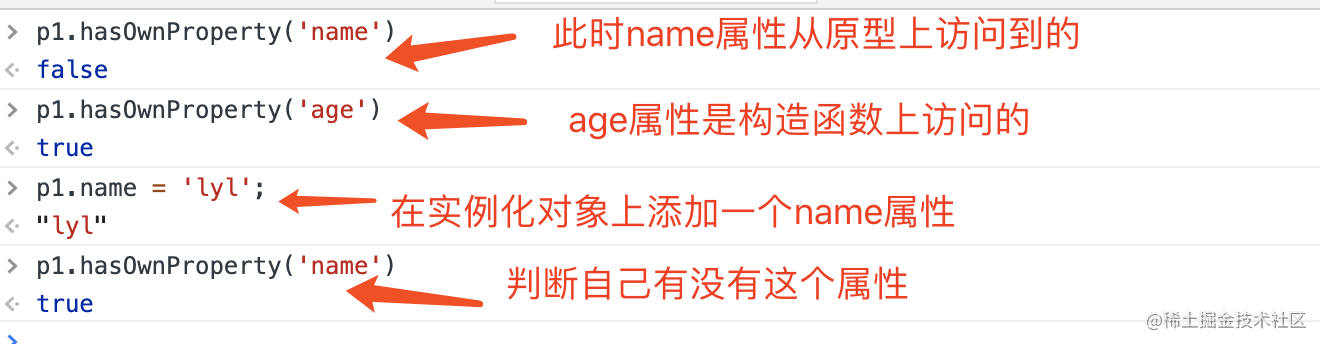in操作符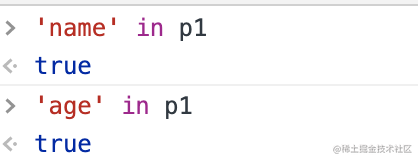更简单的原型语法

function Person(name,age){
this.name = name;
this.age = age;
}
Person.prototype.name = 'yr'
Person.prototype = {
sayName:function(){
console.log(this.name)
},
sayAge:function(){
console.log(this.age)
}
}
let p1 = new Person('yr',86);
let p2 = new Person('lyl',88);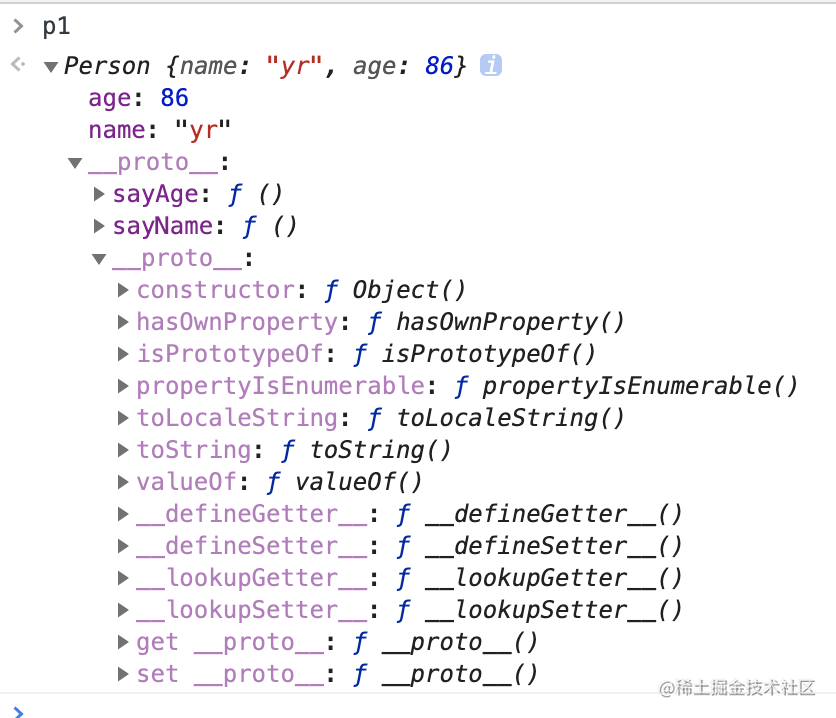Person.prototype = {
constructor:Person,
sayName:function(){
console.log(this.name)
},
sayAge:function(){
console.log(this.age)
}
}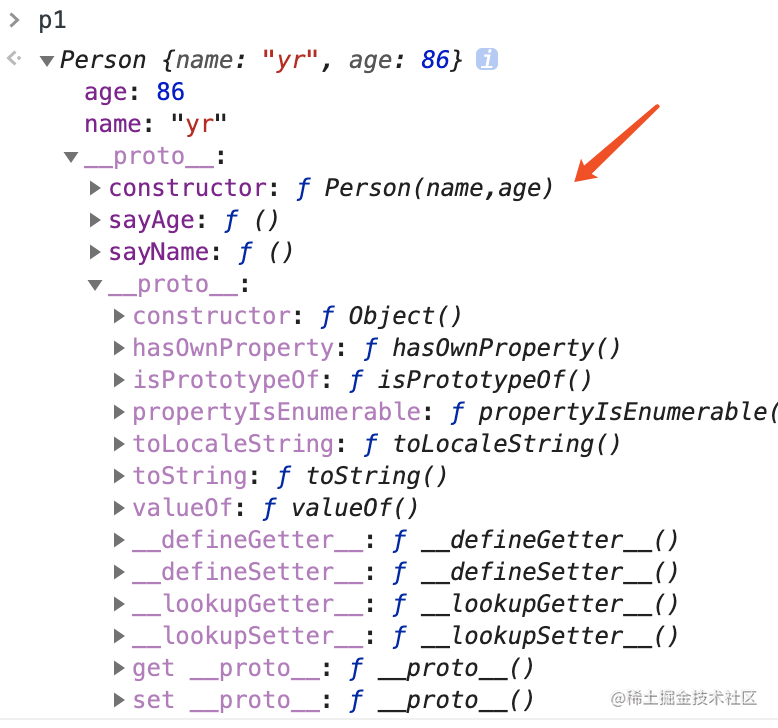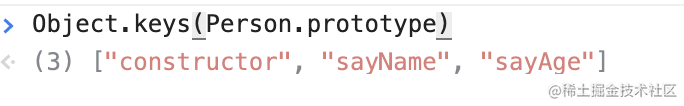function Person(name,age){
this.name = name;
this.age = age;
}
Person.prototype = {
sayName:function(){
console.log(this.name)
},
sayAge:function(){
console.log(this.age)
}
}
Object.defineProperty(Person.prototype,"constructor",{
enumerable:false,
value:Person
})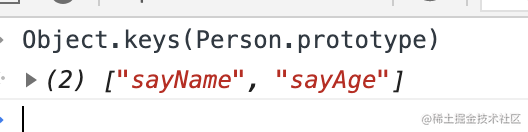function Person(name,age){
this.name = name;
this.age = age;
}
let p1 = new Person('yr',86);
Person.prototype = {
sayName:function(){
console.log(this.name)
},
sayAge:function(){
console.log(this.age)
}
}
Object.defineProperty(Person.prototype,"constructor",{
enumerable:false,
value:Person
})
let p2 = new Person('lyl',88);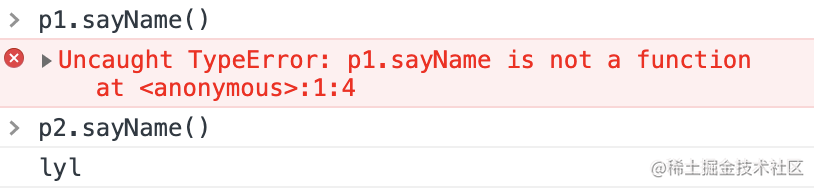原型链